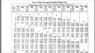# Need help in calculating amount of cold water required

Krishna Swamy
need to cool down 1000 ltrs of water from 121 Degree C to 36 Degree C.Jaket volume of fermentor is 200 ltrs.
How much cold water at 25 Degree C required ?at what flow rate?how much time required.?

Mentor
need to cool down 1000 ltrs of water from 121 Degree C to 36 Degree C.Jaket volume of fermentor is 200 ltrs.
How much cold water at 25 Degree C required ?at what flow rate?how much time required.?

Welcome to the PF.

Water at 121 degrees C is not water...Staff Emeritus
Homework Helper
Welcome to the PF.

Water at 121 degrees C is not water...It can be, if it's under pressure. It seems like the OP has some sort of cooling jacket which operates above the normal B.P. of water.

After all, if you car's engine is water cooled, the cooling system operates above ambient pressure so that the coolant doesn't boil over if its temperature reaches 100 C.

•berkeman
Mentor
...well, that and it contains a decent fraction of glycol...

Is this a one-time temperature drop? Beer/wine or pharmaceutical? Is the vessel pre-engineered? Stirred?

The tough part isn't the cooling requirement itself, but the heat transfer effectiveness.

Staff Emeritus
Homework Helper
...well, that and it contains a decent fraction of glycol...

While glycol addition to coolant will raise the B.P. a tad, that's not why it's used: It's to depress the F.P. of the water, at the other end of the scale, so the engine doesn't pop a freeze plug (or worse).I took out my trusty steam tables, and a pressurized cooling system operating at 1 atm. gauge pressure has a B.P. of almost exactly 120 °C using pure water as the coolant.NickAtNight
mass in fermentor = volume times density. 1000 liters times 1 kg/l = 1000 kg.

exchanger duty required. Q = m Cp dT
T1 = 126 C
T2 = 36 C
DT = T2-T1 = 125 - 36 = 89 C

Cp water = 4.18 kJ/kg K

Q = 1000 kg * 4.18 kJ/Kg K * 89 C = 373,000 kJ

NickAtNight
Cooling water supplied
M = ?
Cp = 4.18 kJ/kg C
T1 = 25 C
Limit water temp rise to 9 C
T2 = 34 C

m = Q / Cp / dT

Q = 373,000 kJ / 4.18 kJ/Kg C / 9 C = 9,915 kg

Let's say you have a 20,000 liter tank that is 1/2 full (10,000 liters of water) and you pump the water around in a loop.

These two equations reduce to m cooling water = liters cooled * (dT cooled / water temp rise) = liters cooled * (89/9)
So you need ten times the water as material being cooled for this assumption.

NickAtNight
A 1000 liter wine fermentor (264 gal) is 41" wide (1000 mm) by 52. Tall ( 1300 mm)

So circumference is pi times D = 3.1416 * 1,000 mm = 3,141.6 mm
Height = 1300 mm
a = C * H = 3,141.6 mm times 1300 mm = 4,084,080 mm^2 = 4 m^2

If the jacket volume is 200 liters is 200,000,000 mm^3, then the jacket is 2" thick (49 mm)

size the exchanger. Q = U A dT log mean

U is complicated... So use a rule of thumb. Jacketed Water Dilute aqueouos solutions 200 W/ m2 C. Where w = J/s

Q/s = 800 J/s * dT. Tinit = 125 C. t final = 36 C. To. T= 25 C going to 34 C
So dT initial is say about 125 C less 30 C = say 100 C
So initial heat loss is about 80,000 J/s

Near the end it will be much slower at. 36 less 30 = 6 c
So about 4,800 J/s at the end

And we have 373,000,000 J to remove

So 22 hours using the slowest rate. 1.5 hour at the fastest rate. Slower rate will dominate so guess about ten hours.

Depends on U.
Thickness of the metal,
Effectiveness of the jacketing contacting the vessel.
If tank is stirred
Materials of construction
Etc, etc, etc

Last edited:
Gold Member
Can you elaborate on your culturing of hyperthermophilic organisms? This sounds like a fascinating area of research.

BoB

NickAtNight
Let's rethink the water side.

Got any details on the jacket design?

At volume 200 liters, I am assuming a 50 mm thick, 3,131.6 mm wide by 1,300 mm tall.

One pass? Spiral wound? Number and size of inlets and outlets?

If the best heat transfer rate is about 80 kJ/s, we can solve for flow. m = Q/Cp dT

m = 80 kJ/s / 4 kJ /kg C * dT

dT. m
10 C. 80/40. 2 kg/s
5 C. 80/20. 4 kg/s
2 C. 80/8. 10 kg/s
1 C. 80/4. 20 kg/s

So what size pump do we have available?

What kind of secondary cooler to reject heat to atm? Then we can have only a small water tank and reservoir

NickAtNight
Well 2 kg/s is 2l/s or 32 U.S. Gal/min or 120 l/min

So we be about 1 m/s (3 ft/s) and 0.1 bar (1.5 psi) / 100 ft of 2" pipe

#### Attachments

•image.jpg
79.7 KB · Views: 599
Last edited: